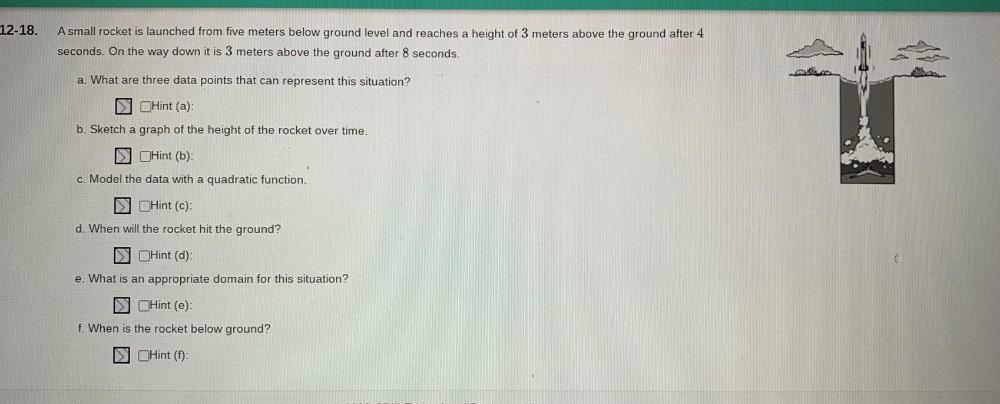Question:

# A small rocket is launched from five meters below ground level and reaches a height of 3 meters above the ground after 4 seconds. On the way down it is 3 meters above the ground after 8 seconds. (a) WA small rocket is launched from five meters below ground level and reaches a height of 3 meters above the ground after 4 seconds. On the way down it is 3 meters above the ground after 8 seconds. (a) What are three data points that can represent this situation? (b) Sketch a graph of the height of the rocket over time. (c) Model the data with a quadratic function. (d) When will the rocket hit the ground? (e) What is an appropriate domain for this situation? (f) When is the rocket below ground?